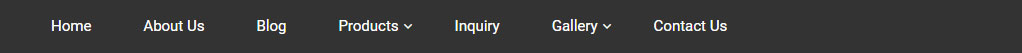Blog Detail# Page TitleMay 28,2022

# 5 Debt Mutual Fund Terms That You Need to Know Before Investing

With the low-interest rates on traditional saving instruments, many individuals are looking at different investment options to protect their capital and earn stable returns. Debt mutual funds are one such investment option that has gained popularity among individual investors in the last few years.

However, debt funds are a little complicated than equity funds, and many of the terms associated with debt mutual funds may sound like Greek to many investors. So, today we have collated a list of essential terms that you need to be aware of before investing in debt mutual funds.

## Coupon rate

The coupon on a bond is the annual interest rate paid by the bond's issuer. For example, if a company is issuing bonds, the coupon rate will be the interest rate they will pay to their investors. The issuer company might pay the coupon on bonds quarterly, semi-annually, or annually.

Let's say you put Rs 5,000 into a 6-year bond with a coupon rate of 5% per year, paid semi-annually.

Annual coupon payment = 5%*5000 = 250

Per coupon payment = 250/2

## Yield

Many investors confuse the coupon rate with yield. But it is not the same. Let's assume that a bond has a face value of Rs 100 with a 6% coupon rate. This indicates that for each bond purchased, the investor will receive Rs 7 every year.

However, once the bond is issued, it trades on the open market just like a stock. So, the price of the bond will fluctuate until its maturity date. The bond price will fluctuate as interest rates in the economy rise and fall, and demand for bonds rises and falls.

Assume that interest rates increase to 10%. However, the investor, in case the debt mutual fund, will continue to earn Rs 8. So, to raise the yield to 10%, which is the current market rate of interest, the bond's price will have to fall to Rs 80. So, we have seen that the yield is not constant, and the bond's price moves in the opposite direction of interest rates. So, an unexpected hike in interest rate follows a sharp fall in debt fund returns.

## Yield to Maturity (YTM)

The YTM of a debt mutual fund is the expected rate of return, assuming that the fund manager holds all the securities in the portfolio to maturity. Taking a debt fund has a YTM of 10%, for example, it means that if the portfolio remains unchanged until all the securities mature, the investor will receive a 10% return. However, the YTM does not remain constant because the fund manager actively manages the portfolios.

Yield to maturity gives the debt fund investor a general idea of the returns they can expect. Returns may fluctuate owing to mark-to-market values or changes in the portfolio. Therefore, it's not a sure thing.

## Modified Duration

Modified duration refers to the change in the value of debt instruments, such as bonds, because of interest rate changes. Let's assume the bond's modified duration is 4.50. So, with a 1% increase in interest rates, the bond's price will fall by 4.50%.

This gives a good idea of how sensitive a bond is to interest rate changes.

So, a debt instrument with a higher modified duration will be more volatile during a change in interest rate than an instrument with a lower modified duration. Because the modified duration of a portfolio considers all debt instruments, it will alter depending on the portfolio's composition.

## Weighted Average Maturity

This is mostly referred to as a debt fund's average maturity. The weighted average maturity is the average maturity period of all the securities in a debt mutual fund after considering the invested amount.

For example, Rs 1,000 investment in Bond A will mature after five years.

Bond B, which costs Rs 2,000, matures in ten years.

Total loan portfolio investment = Rs 3,000 WAM = 1000/3000*5 + 2000/3000*10 = 8.33 years

The average maturity of a portfolio reveals how sensitive it is to interest rate changes. The higher the average maturity, the more volatile the fund's returns are. So, debt funds with long-duration bonds are more volatile than liquid funds that need to invest in debt instruments with a maximum maturity of 90 days.

The average maturity provides a general guideline for selecting a debt fund that is appropriate for your investing time horizon.

Conclusion: Coupon rate, yield, yield to maturity, modified duration and weighted average maturity are the key terms you need to learn before investing in debt mutual funds.

This blog is purely for educational purposes and not to be treated as personal advice. Mutual fund investments are subject to market risks, read all scheme related documents carefully.

Tags :

All Blog